# MetaTrader 5 中的多元品种余额图

25 五月 2018, 16:55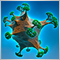0
12 302

### 开发图形界面

• 控件的窗体。
• 用最后一次测试结果来更新图表的按钮。
• 多元品种余额图。
• 资金回撤图。
• 用来显示额外摘要信息的状态栏。

```//+------------------------------------------------------------------+
//| 用于创建应用程序的类                                                 |
//+------------------------------------------------------------------+
class CProgram : public CWndEvents
{
private:
//--- 窗体
CWindow           m_window1;
//--- 状态栏
CStatusBar        m_status_bar;
//--- 图形
CGraph            m_graph1;
CGraph            m_graph2;
//--- 按钮
CButton           m_update_graph;
//---
public:
//--- 创建图形界面
bool              CreateGUI(void);
//---
private:
//--- 窗体
bool              CreateWindow(const string text);
//--- 状态栏
bool              CreateStatusBar(const int x_gap,const int y_gap);
//--- 图形
bool              CreateGraph1(const int x_gap,const int y_gap);
bool              CreateGraph2(const int x_gap,const int y_gap);
//--- 按钮
bool              CreateUpdateGraph(const int x_gap,const int y_gap,const string text);
};
//+------------------------------------------------------------------+
//| 创建控件元素的方法                                                   |
//+------------------------------------------------------------------+
#include "CreateGUI.mqh"
//+------------------------------------------------------------------+
```

```//+------------------------------------------------------------------+
//| 创建图形界面                                                       |
//+------------------------------------------------------------------+
bool CProgram::CreateGUI(void)
{
//--- 为控件元素创建窗体
if(!CreateWindow("Expert panel"))
return(false);
//--- 创建控件元素
if(!CreateStatusBar(1,23))
return(false);
if(!CreateGraph1(1,50))
return(false);
if(!CreateGraph2(1,159))
return(false);
if(!CreateUpdateGraph(7,25,"Update data"))
return(false);
//--- 图形界面创建完毕
CWndEvents::CompletedGUI();
return(true);
}
```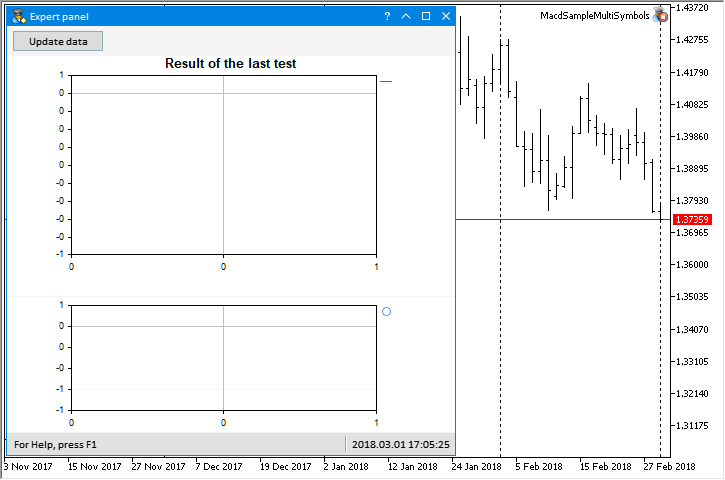### 测试的多元品种 EA

```//--- 外部参数
sinput string Symbols           ="EURUSD,USDJPY,GBPUSD,EURCHF"; // 品种
input  double InpLots           =0.1;                           // 手数
input  int    InpTakeProfit     =167;                           // 止盈 (点数)
input  int    InpTrailingStop   =97;                            // 尾随停止级别 (点数)
input  int    InpMACDOpenLevel  =16;                            // MACD 开仓级别 (点数)
input  int    InpMACDCloseLevel =19;                            // MACD 平仓级别 (点数)
input  int    InpMATrendPeriod  =14;                            // MA 趋势周期
```

```class CProgram : public CWndEvents
{
private:
//--- 检查所传递数组中的交易品种并返回可用数组之一
void              CheckTradeSymbols(string &source_array[],string &checked_array[]);
};
//+------------------------------------------------------------------+
//| 检查所传递数组中的交易品种                                            |
//| 并返回可用数组之一                                                   |
//+------------------------------------------------------------------+
void CProgram::CheckTradeSymbols(string &source_array[],string &checked_array[])
{
int symbols_total     =::SymbolsTotal(false);
int size_source_array =::ArraySize(source_array);
//--- 在总列表中查找指定的品种
for(int i=0; i<size_source_array; i++)
{
for(int s=0; s<symbols_total; s++)
{
//--- 获取公共列表中当前品种的名称
string symbol_name=::SymbolName(s,false);
//--- 如果匹配
if(symbol_name==source_array[i])
{
//--- 在市场观察中设置一个品种
::SymbolSelect(symbol_name,true);
//--- 添加到确认的品种数组
int size_array=::ArraySize(checked_array);
::ArrayResize(checked_array,size_array+1);
checked_array[size_array]=symbol_name;
break;
}
}
}
//--- 如果未检测到品种，则仅使用当前品种
if(::ArraySize(checked_array)<1)
{
::ArrayResize(checked_array,1);
checked_array=_Symbol;
}
}
```

CProgram::CheckSymbols() 方法用于读取外部字符串参数中指定的品种。 此处，字符串被切分为一个数组，用 ',' 作为分隔符。 彼此间的间隙都是由此产生的。 之后，数组 将被发送给上面曾研究过的 CProgram::CheckTradeSymbols() 方法进行验证。

```class CProgram : public CWndEvents
{
private:
//--- 检查来自字符串的交易品种并包含到数组
int               CheckSymbols(const string symbols_enum);
};
//+-------------------------------------------------------------------------+
//| 检查来自字符串的交易品种并包含到数组                                           |
//+-------------------------------------------------------------------------+
int CProgram::CheckSymbols(const string symbols_enum)
{
if(symbols_enum!="")
::Print(__FUNCTION__," > 输入交易品种: ",symbols_enum);
//--- 从字符串中获取品种
string symbols[];
ushort u_sep=::StringGetCharacter(",",0);
::StringSplit(symbols_enum,u_sep,symbols);
//--- 从两侧剔除空白
int elements_total=::ArraySize(symbols);
for(int e=0; e<elements_total; e++)
{
::StringTrimLeft(symbols[e]);
::StringTrimRight(symbols[e]);
}
//--- 检查品种
::ArrayFree(m_symbols);
CheckTradeSymbols(symbols,m_symbols);
//--- 获取交易品种的数量
return(::ArraySize(m_symbols));
}
```

```#include "Strategy.mqh"
//+------------------------------------------------------------------+
//| 用于创建应用程序的类                                                 |
//+------------------------------------------------------------------+
class CProgram : public CWndEvents
{
private:
//--- 策略数组
CStrategy         m_strategy[];
};
```

```class CProgram : public CWndEvents
{
private:
//--- 品种总数
int               m_symbols_total;
};
//+------------------------------------------------------------------+
//| 初始化                                                            |
//+------------------------------------------------------------------+
bool CProgram::OnInitEvent(void)
{
//--- 获取交易品种
m_symbols_total=CheckSymbols(Symbols);
//--- 调整数组大小
::ArrayResize(m_strategy,m_symbols_total);
//--- 初始化
for(int i=0; i<m_symbols_total; i++)
{
if(!m_strategy[i].OnInitEvent(m_symbols[i]))
return(false);
}
//--- 初始化成功
return(true);
}
```

### 将数据写入文件

```class CProgram : public CWndEvents
{
private:
//--- 最后的测试结果的保存文件路径
string            m_last_test_report_path;
};
//+------------------------------------------------------------------+
//| 构造器                                                            |
//+------------------------------------------------------------------+
CProgram::CProgram(void) : m_symbols_total(0)
{
//--- 最后的测试结果的保存文件路径
m_last_test_report_path=::MQLInfoString(MQL_PROGRAM_NAME)+"\\LastTest.csv";
}
```

```//--- 所有品种的余额数组
struct CReportBalance { double m_data[]; };
//+------------------------------------------------------------------+
//| 用于创建应用程序的类                                                 |
//+------------------------------------------------------------------+
class CProgram : public CWndEvents
{
private:
//--- 所有品种的余额数组
CReportBalance    m_symbol_balance[];
//---
private:
//--- 创建 CSV 格式的交易测试报告
void              CreateSymbolBalanceReport(void);
};
//+------------------------------------------------------------------+
//| 创建 CSV 格式的交易测试报告                                          |
//+------------------------------------------------------------------+
void CProgram::CreateSymbolBalanceReport(void)
{
...
}
```

```...
//--- 在终端公共文件夹中创建一个用于写入数据的文件
int file_handle=::FileOpen(m_last_test_report_path,FILE_CSV|FILE_WRITE|FILE_ANSI|FILE_COMMON);
//--- 如果句柄有效 (文件已创建/已打开)
if(file_handle==INVALID_HANDLE)
{
::Print(__FUNCTION__," > 创建文件错误: ",::GetLastError());
return;
}
...
```

• 成交时间
• 品种
• 类型
• 方向
• 交易量
• 价格
• 掉期利率
• 结果 (盈利/亏损)
• 回撤
• 余额。 此列显示余额总数，而后面的列包含测试中使用的各品种余额

```...
double max_drawdown    =0.0; // 最大回撤
double balance         =0.0; // 余额
string delimeter       =","; // 分隔符
string string_to_write ="";  // 为了形成一个入场行
//--- 形成标题行
string headers="TIME,SYMBOL,DEAL TYPE,ENTRY TYPE,VOLUME,PRICE,SWAP(\$),PROFIT(\$),DRAWDOWN(%),BALANCE";
...
```

```...
//--- 如果涉及多元品种，则补充标题行
int symbols_total=::ArraySize(m_symbols);
if(symbols_total>1)
{
for(int s=0; s<symbols_total; s++)
::StringAdd(headers,delimeter+m_symbols[s]);
}
//--- 写报告标题
::FileWrite(file_handle,headers);
...
```

```...
//--- 获取整个历史
::HistorySelect(0,LONG_MAX);
//--- 找出成交数量
int deals_total=::HistoryDealsTotal();
//--- 通过品种数量设置余额数组的数量
::ArrayResize(m_symbol_balance,symbols_total);
//--- 为每个品种设置成交数组的大小
for(int s=0; s<symbols_total; s++)
::ArrayResize(m_symbol_balance[s].m_data,deals_total);
...
```

```...
//--- 沿循环移动并写入数据
for(int i=0; i<deals_total; i++)
{
//--- 获得成交单号
if(!m_deal_info.SelectByIndex(i))
continue;
//--- 找出价格中的小数位数
int digits=(int)::SymbolInfoInteger(m_deal_info.Symbol(),SYMBOL_DIGITS);
//--- 计算总余额
balance+=m_deal_info.Profit()+m_deal_info.Swap()+m_deal_info.Commission();
//--- 形成连接写入的行
::StringConcatenate(string_to_write,
::TimeToString(m_deal_info.Time(),TIME_DATE|TIME_MINUTES),delimeter,
m_deal_info.Symbol(),delimeter,
m_deal_info.TypeDescription(),delimeter,
m_deal_info.EntryDescription(),delimeter,
::DoubleToString(m_deal_info.Volume(),2),delimeter,
::DoubleToString(m_deal_info.Price(),digits),delimeter,
::DoubleToString(m_deal_info.Swap(),2),delimeter,
::DoubleToString(m_deal_info.Profit(),2),delimeter,
MaxDrawdownToString(i,balance,max_drawdown),delimeter,
::DoubleToString(balance,2));
//--- 如果有多元品种，请写入其余额值
if(symbols_total>1)
{
//--- 沿着所有品种移动
for(int s=0; s<symbols_total; s++)
{
//--- 如果品种匹配并且成交结果不为零
if(m_deal_info.Symbol()==m_symbols[s] && m_deal_info.Profit()!=0)
//--- 显示此品种一笔交易的余额。 考虑掉期利率和佣金
m_symbol_balance[s].m_data[i]=m_symbol_balance[s].m_data[i-1]+m_deal_info.Profit()+m_deal_info.Swap()+m_deal_info.Commission();
//--- 否则，写入之前的值
else
{
//--- 如果是 "资金余额" 交易 (第一笔交易)，所有品种的余额是相同的
if(m_deal_info.DealType()==DEAL_TYPE_BALANCE)
m_symbol_balance[s].m_data[i]=balance;
//--- 否则，将以前的值写入当前索引
else
m_symbol_balance[s].m_data[i]=m_symbol_balance[s].m_data[i-1];
}
//--- 将品种余额添加到字符串
::StringAdd(string_to_write,delimeter+::DoubleToString(m_symbol_balance[s].m_data[i],2));
}
}
//--- 写入形成的字符串
::FileWrite(file_handle,string_to_write);
//--- 强制将下一个字符串的变量设置为零
string_to_write="";
}
//--- 关闭文件
::FileClose(file_handle);
...
```

```class CProgram : public CWndEvents
{
private:
//--- 从局部最大值获得最大回撤
string            MaxDrawdownToString(const int deal_number,const double balance,double &max_drawdown);
};
//+------------------------------------------------------------------+
//| 从局部最大值获得最大回撤                                              |
//+------------------------------------------------------------------+
string CProgram::MaxDrawdownToString(const int deal_number,const double balance,double &max_drawdown)
{
//--- 用于在报告中显示的字符串
string str="";
//--- 用于局部最大和回撤计算
static double max=0.0;
static double min=0.0;
//--- 如果是首笔交易
if(deal_number==0)
{
//--- 尚无回撤
max_drawdown=0.0;
//--- 将初始点设置为局部最大值
max=balance;
min=balance;
}
else
{
//--- 如果当前余额超过保存的余额
if(balance>max)
{
//--- 按先前的值计算回撤
max_drawdown=100-((min/max)*100);
//--- 更新本地最大值
max=balance;
min=balance;
}
else
{
//--- 获得零回撤并更新最小值
max_drawdown=0.0;
min=fmin(min,balance);
}
}
//--- 为报告定义字符串
str=(max_drawdown==0)? "" : ::DoubleToString(max_drawdown,2);
return(str);
}
```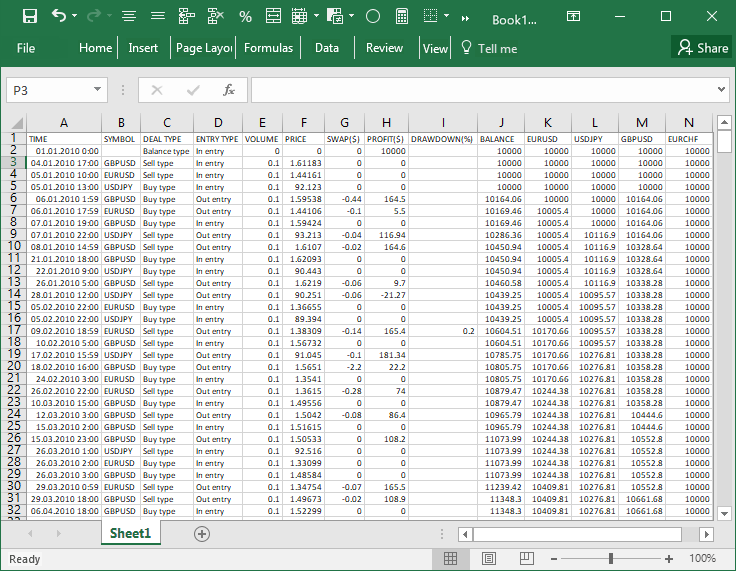```//+------------------------------------------------------------------+
//| 测试完成事件                                                       |
//+------------------------------------------------------------------+
double CProgram::OnTesterEvent(void)
{
//--- 只有在测试后才写入报告
if(::MQLInfoInteger(MQL_TESTER) && !::MQLInfoInteger(MQL_OPTIMIZATION) &&
!::MQLInfoInteger(MQL_VISUAL_MODE) && !::MQLInfoInteger(MQL_FRAME_MODE))
{
//--- 形成报告并写入文件
CreateSymbolBalanceReport();
}
//---
return(0.0);
}
```

### 从文件中提取数据

```class CProgram : public CWndEvents
{
private:
//--- 来自文件数据的数组
string            m_source_data[];
//---
private:
//--- 将文件读取到传递的数组
bool              ReadFileToArray(const int file_handle);
};
//+------------------------------------------------------------------+
//| 将文件读取到传递的数组                                               |
//+------------------------------------------------------------------+
bool CProgram::ReadFileToArray(const int file_handle)
{
//--- 打开文件
int file_handle=::FileOpen(m_last_test_report_path,FILE_READ|FILE_ANSI|FILE_COMMON);
//--- 如果文件尚未打开，则退出
if(file_handle==INVALID_HANDLE)
return(false);
//--- 释放数组
::ArrayFree(m_source_data);
//--- 将文件读取到数组
while(!::FileIsEnding(file_handle))
{
int size=::ArraySize(m_source_data);
::ArrayResize(m_source_data,size+1,RESERVE);
m_source_data[size]=::FileReadString(file_handle);
}
//--- 关闭文件
::FileClose(file_handle);
return(true);
}
```

```class CProgram : public CWndEvents
{
private:
//--- 报告中的初始 baLalnce 指数
bool              GetBalanceIndex(const string headers);
};
//+------------------------------------------------------------------+
//| 定义开始复制数据的索引                                               |
//+------------------------------------------------------------------+
bool CProgram::GetBalanceIndex(const string headers)
{
//--- 通过分隔符获取字符串元素
string str_elements[];
ushort u_sep=::StringGetCharacter(",",0);
::StringSplit(headers,u_sep,str_elements);
//--- 搜索 'BALANCE' 列
int elements_total=::ArraySize(str_elements);
for(int e=elements_total-1; e>=0; e--)
{
string str=str_elements[e];
::StringToUpper(str);
//--- 如果找到含有必要标题的列
if(str=="BALANCE")
{
m_balance_index=e;
break;
}
}
//--- 如果找不到 'BALANCE' 列，则显示消息
if(m_balance_index==WRONG_VALUE)
{
::Print(__FUNCTION__," > 在报告文件里, 未有 \'BALANCE\' 题头! ");
return(false);
}
//--- 成功
return(true);
}
```

```class CProgram : public CWndEvents
{
private:
//--- 日期范围
void              GetDateRange(string &from_date,string &to_date);
};
//+------------------------------------------------------------------+
//| 获取测试范围的开始和结束日期                                          |
//+------------------------------------------------------------------+
void CProgram::GetDateRange(string &from_date,string &to_date)
{
//--- 如果少于三个字符串则退出
int strings_total=::ArraySize(m_source_data);
if(strings_total<3)
return;
//--- 获取报告的开始和结束日期
string str_elements[];
ushort u_sep=::StringGetCharacter(",",0);
//---
::StringSplit(m_source_data,u_sep,str_elements);
from_date=str_elements;
::StringSplit(m_source_data[strings_total-1],u_sep,str_elements);
to_date=str_elements;
}
```

CProgram::GetReportDataToArray() 和 CProgram::AddDrawDown() 方法用于获取余额和回撤数据。 第二个调用第一个，它的代码非常短 (见下面的清单)。 此处传递交易指数和回撤值。 将索引和值插入适当的数组中，然后将其值显示在图上。 绘制的值保存在 m_dd_y[]，而显示此值的索引保存在 m_dd_x[]。 因此，索引处没有数值则在图上不显示任何内容 (空值)。

```class CProgram : public CWndEvents
{
private:
//--- 总余额回撤
double            m_dd_x[];
double            m_dd_y[];
//---
private:
//--- 将回撤添加到数组中
void              AddDrawDown(const int index,const double drawdown);
};
//+------------------------------------------------------------------+
//| 将回撤添加到数组中                                                   |
//+------------------------------------------------------------------+
void CProgram::AddDrawDown(const int index,const double drawdown)
{
int size=::ArraySize(m_dd_y);
::ArrayResize(m_dd_y,size+1,RESERVE);
::ArrayResize(m_dd_x,size+1,RESERVE);
m_dd_y[size] =drawdown;
m_dd_x[size] =(double)index;
}
```

```class CProgram : public CWndEvents
{
private:
//--- 从报告中获取品种数据
int               GetReportDataToArray(string &headers[]);
};
//+------------------------------------------------------------------+
//| 从报告中获取品种数据                                                 |
//+------------------------------------------------------------------+
int CProgram::GetReportDataToArray(string &headers[])
{
//--- 获取标题字符串元素
string str_elements[];
ushort u_sep=::StringGetCharacter(",",0);
::StringSplit(m_source_data,u_sep,str_elements);
//--- 数组大小
int strings_total  =::ArraySize(m_source_data);
int elements_total =::ArraySize(str_elements);
//--- 释放数组
::ArrayFree(m_dd_y);
::ArrayFree(m_dd_x);
//--- 获取序列的数量
int curves_total=elements_total-m_balance_index;
curves_total=(curves_total<3)? 1 : curves_total;
//--- 按序列数量设置数组的大小
::ArrayResize(headers,curves_total);
::ArrayResize(m_symbol_balance,curves_total);
//--- 设置序列的大小
for(int i=0; i<curves_total; i++)
::ArrayResize(m_symbol_balance[i].m_data,strings_total,RESERVE);
//--- 如果有若干个品种 (接题头)
if(curves_total>2)
{
for(int i=0,e=m_balance_index; e<elements_total; e++,i++)
headers[i]=str_elements[e];
}
else
headers=str_elements[m_balance_index];
//--- 获取数据
for(int i=1; i<strings_total; i++)
{
::StringSplit(m_source_data[i],u_sep,str_elements);
//--- 收集数据至数组
if(str_elements[m_balance_index-1]!="")
AddDrawDown(i,double(str_elements[m_balance_index-1]));
//--- 如果有若干个品种
if(curves_total>2)
for(int b=0,e=m_balance_index; e<elements_total; e++,b++)
m_symbol_balance[b].m_data[i]=double(str_elements[e]);
else
m_symbol_balance.m_data[i]=double(str_elements[m_balance_index]);
}
//--- 第一个序列的值
for(int i=0; i<curves_total; i++)
m_symbol_balance[i].m_data=(strings_total<2)? 0 : m_symbol_balance[i].m_data;
//--- 获取序列的数量
return(curves_total);
}
```

### 在图形上显示数据

```class CProgram : public CWndEvents
{
private:
//--- 序列中的数据总数
double            m_data_total;
//--- X 轴上的刻度间距
double            m_default_step;
//---
private:
//--- 更新余额图上的数据
void              UpdateBalanceGraph(void);
};
//+------------------------------------------------------------------+
//| 更新余额图                                                         |
//+------------------------------------------------------------------+
void CProgram::UpdateBalanceGraph(void)
{
//--- 获取测试范围日期
string from_date=NULL,to_date=NULL;
GetDateRange(from_date,to_date);
//--- 定义开始复制数据的索引
if(!GetBalanceIndex(m_source_data))
return;
//--- 从报告中获取品种数据
string headers[];
int curves_total=GetReportDataToArray(headers);

//--- 使用新数据更新所有图表序列
CColorGenerator m_generator;
CGraphic *graph=m_graph1.GetGraphicPointer();
//--- 清除图表
int total=graph.CurvesTotal();
for(int i=total-1; i>=0; i--)
graph.CurveRemoveByIndex(i);
//--- 图表高点和低点
double y_max=0.0,y_min=m_symbol_balance.m_data;
//--- 添加数据
for(int i=0; i<curves_total; i++)
{
//--- 定义 Y 轴的高点/低点
y_max=::fmax(y_max,m_symbol_balance[i].m_data[::ArrayMaximum(m_symbol_balance[i].m_data)]);
y_min=::fmin(y_min,m_symbol_balance[i].m_data[::ArrayMinimum(m_symbol_balance[i].m_data)]);
//--- 将序列添加到图表
CCurve *curve=graph.CurveAdd(m_symbol_balance[i].m_data,m_generator.Next(),CURVE_LINES,headers[i]);
}
//--- 数值数量和 X 轴网格步长
m_data_total   =::ArraySize(m_symbol_balance.m_data)-1;
m_default_step =(m_data_total<10)? 1 : ::MathFloor(m_data_total/5.0);
//--- 范围和缩进
double range  =::fabs(y_max-y_min);
double offset =range*0.05;
//--- 第一个序列的颜色
graph.CurveGetByIndex(0).Color(::ColorToARGB(clrCornflowerBlue));
//--- 横轴属性
CAxis *x_axis=graph.XAxis();
x_axis.AutoScale(false);
x_axis.Min(0);
x_axis.Max(m_data_total);
x_axis.MaxGrace(0);
x_axis.MinGrace(0);
x_axis.DefaultStep(m_default_step);
x_axis.Name(from_date+" - "+to_date);
//--- 纵轴属性
CAxis *y_axis=graph.YAxis();
y_axis.AutoScale(false);
y_axis.Min(y_min-offset);
y_axis.Max(y_max+offset);
y_axis.MaxGrace(0);
y_axis.MinGrace(0);
y_axis.DefaultStep(range/10.0);
//--- 更新图形
graph.CurvePlotAll();
graph.Update();
}
```

CProgram::UpdateDrawdownGraph() 方法用于更新回撤图。 由于数据已在 CProgram::UpdateBalanceGraph() 方法中计算，此处我们仅需应用它们并刷新图形。

```class CProgram : public CWndEvents
{
private:
//--- 更新回撤图上的数据
void              UpdateDrawdownGraph(void);
};
//+------------------------------------------------------------------+
//| 更新回撤图                                                         |
//+------------------------------------------------------------------+
void CProgram::UpdateDrawdownGraph(void)
{
//--- 更新回撤图
CGraphic *graph=m_graph2.GetGraphicPointer();
CCurve *curve=graph.CurveGetByIndex(0);
curve.Update(m_dd_x,m_dd_y);
curve.PointsFill(false);
curve.PointsSize(6);
curve.PointsType(POINT_CIRCLE);
//--- 横轴属性
CAxis *x_axis=graph.XAxis();
x_axis.AutoScale(false);
x_axis.Min(0);
x_axis.Max(m_data_total);
x_axis.MaxGrace(0);
x_axis.MinGrace(0);
x_axis.DefaultStep(m_default_step);
//--- 更新图形
graph.CalculateMaxMinValues();
graph.CurvePlotAll();
graph.Update();
}
```

CProgram::UpdateBalanceGraph() 和 CProgram::UpdateDrawdownGraph() 方法在 CProgram::UpdateGraphs() 方法中调用。 在调用它们之前，首先调用 CProgram::ReadFileToArray() 方法。 它从文件里接收 EA 上次测试结果数据。

```class CProgram : public CWndEvents
{
private:
//--- 更新最后测试结果图上的数据
void              UpdateGraphs(void);
};
//+------------------------------------------------------------------+
//| 更新图形                                                           |
//+------------------------------------------------------------------+
void CProgram::UpdateGraphs(void)
{
//--- 用文件中的数据填充数组
if(!ReadFileToArray())
{
::Print(__FUNCTION__," > 无法打开测试结果文件!");
return;
}
//--- 刷新余额和回撤图
UpdateBalanceGraph();
UpdateDrawdownGraph();
}
```

### 显示获得的结果

```//+------------------------------------------------------------------+
//| 事件处理器                                                         |
//+------------------------------------------------------------------+
void CProgram::OnEvent(const int id,const long &lparam,const double &dparam,const string &sparam)
{
//--- 按钮点击事件
if(id==CHARTEVENT_CUSTOM+ON_CLICK_BUTTON)
{
//--- 按下 '更新数据'
if(lparam==m_update_graph.Id())
{
//--- 更新图形
UpdateGraphs();
return;
}
//---
return;
}
}
```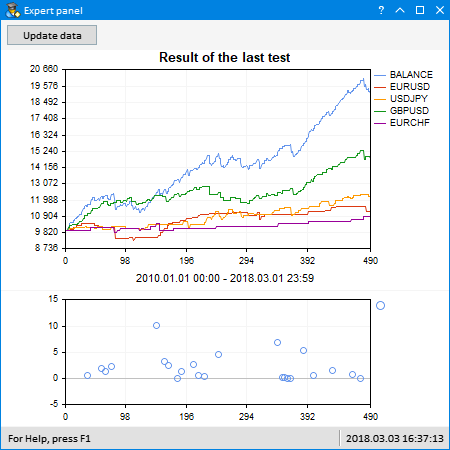### 交易和测试期间的多元品种余额图

```class CProgram : public CWndEvents
{
private:
//--- 最后更改的成交时间和单号
datetime          m_last_deal_time;
ulong             m_last_deal_ticket;
//---
private:
//--- 检查新成交
bool              IsLastDealTicket(void);
};
//+------------------------------------------------------------------+
//| 构造器                                                            |
//+------------------------------------------------------------------+
CProgram::CProgram(void) : m_last_deal_time(NULL),
m_last_deal_ticket(WRONG_VALUE)
{
}
//+------------------------------------------------------------------+
//| 获取指定品种的最后成交事件                                            |
//+------------------------------------------------------------------+
bool CProgram::IsLastDealTicket(void)
{
//--- 如果尚未收到，则退出
if(!::HistorySelect(m_last_deal_time,LONG_MAX))
return(false);
//--- 从获取的列表中得到成交数量
int total_deals=::HistoryDealsTotal();
//--- 从尾至头遍历接收列表中的所有成交
for(int i=total_deals-1; i>=0; i--)
{
//--- 获得成交单号
ulong deal_ticket=::HistoryDealGetTicket(i);
//--- 如果单号相同，退出
if(deal_ticket==m_last_deal_ticket)
return(false);
//--- 如果单号不相同，通知
else
{
datetime deal_time=(datetime)::HistoryDealGetInteger(deal_ticket,DEAL_TIME);
//--- 保存最后一笔成交的时间和单号
m_last_deal_time   =deal_time;
m_last_deal_ticket =deal_ticket;
return(true);
}
}
//--- 另一个品种的单号
return(false);
}

```

```class CProgram : public CWndEvents
{
private:
//--- 来自历史的品种数组
string            m_symbols_name[];
//---
private:
//--- 从帐户历史记录中获取品种并返回它们的数量
int               GetHistorySymbols(void);
};
//+------------------------------------------------------------------+
//| 从帐户历史记录中获取品种并返回它们的数量                                 |
//+------------------------------------------------------------------+
int CProgram::GetHistorySymbols(void)
{
string check_symbols="";
//--- 首次循环遍历并获得交易的品种
int deals_total=::HistoryDealsTotal();
for(int i=0; i<deals_total; i++)
{
//--- 获得成交单号
if(!m_deal_info.SelectByIndex(i))
continue;
//--- 如果有品种名称
if(m_deal_info.Symbol()=="")
continue;
//--- 如果没有这样的字符串，则添加它
if(::StringFind(check_symbols,m_deal_info.Symbol(),0)==-1)
::StringAdd(check_symbols,(check_symbols=="")? m_deal_info.Symbol() : ","+m_deal_info.Symbol());
}
//--- 按分隔符获取字符串元素
ushort u_sep=::StringGetCharacter(",",0);
int symbols_total=::StringSplit(check_symbols,u_sep,m_symbols_name);
//--- 返回品种的数量
return(symbols_total);
}

```

```class CProgram : public CWndEvents
{
private:
//--- 分别为每个品种获取余额总数和余额
void              GetHistorySymbolsBalance(void);
};
//+------------------------------------------------------------------+
//| 分别为每个品种获取总余额和余额                                         |
//+------------------------------------------------------------------+
void CProgram::GetHistorySymbolsBalance(void)
{
...
}
```

```...
//--- 初始存款金额
::HistorySelect(0,LONG_MAX);
double balance=(m_deal_info.SelectByIndex(0))? m_deal_info.Profit() : 0;
//--- 从指定的日期获取历史记录
::HistorySelect(m_from_trade.SelectedDate(),LONG_MAX);
//--- 获取品种的数量
int symbols_total=GetHistorySymbols();
//--- 释放数组
::ArrayFree(m_dd_x);
::ArrayFree(m_dd_y);
//--- 为总余额设置余额数组的大小为品种数 + 1
::ArrayResize(m_symbols_balance,(symbols_total>1)? symbols_total+1 : 1);
//--- 设置每个品种成交数组的大小
int deals_total=::HistoryDealsTotal();
for(int s=0; s<=symbols_total; s++)
{
if(symbols_total<2 && s>0)
break;
//---
::ArrayResize(m_symbols_balance[s].m_data,deals_total);
::ArrayInitialize(m_symbols_balance[s].m_data,0);
}
//--- 余额曲线的数量
int balances_total=::ArraySize(m_symbols_balance);
//--- 历史记录的开始和结束
m_begin_date =(m_deal_info.SelectByIndex(0))? m_deal_info.Time() : m_from_trade.SelectedDate();
m_end_date   =(m_deal_info.SelectByIndex(deals_total-1))? m_deal_info.Time() : ::TimeCurrent();
...
```

```...
//--- 最大回撤
double max_drawdown=0.0;
//--- 将余额数组写入传递的数组
for(int i=0; i<deals_total; i++)
{
//--- 获取成交数组
if(!m_deal_info.SelectByIndex(i))
continue;
//--- 初始化首笔交易
if(i==0 && m_deal_info.DealType()==DEAL_TYPE_BALANCE)
balance=0;
//--- 从指定日期开始
if(m_deal_info.Time()>=m_from_trade.SelectedDate())
{
//--- 计算总余额
balance+=m_deal_info.Profit()+m_deal_info.Swap()+m_deal_info.Commission();
m_symbols_balance.m_data[i]=balance;
//--- 计算回撤
if(MaxDrawdownToString(i,balance,max_drawdown)!="")
AddDrawDown(i,max_drawdown);
}
//--- 如果使用多元品种，则写入品种的余额值
if(symbols_total<2)
continue;
//--- 仅从指定日期
if(m_deal_info.Time()<m_from_trade.SelectedDate())
continue;
//--- 遍历所有品种
for(int s=1; s<balances_total; s++)
{
int prev_i=i-1;
//--- 如果是 "余额存款" 交易 (首笔交易) ...
if(prev_i<0 || m_deal_info.DealType()==DEAL_TYPE_BALANCE)
{
//--- ... 所有品种的余额是相同的
m_symbols_balance[s].m_data[i]=balance;
continue;
}
//--- 如果品种相同且交易结果不为零
if(m_deal_info.Symbol()==m_symbols_name[s-1] && m_deal_info.Profit()!=0)
{
//--- 在余额中反映这一品种的成交。 考虑掉期利率和佣金。
m_symbols_balance[s].m_data[i]=m_symbols_balance[s].m_data[prev_i]+m_deal_info.Profit()+m_deal_info.Swap()+m_deal_info.Commission();
}
//--- 否则，写入之前的值
else
m_symbols_balance[s].m_data[i]=m_symbols_balance[s].m_data[prev_i];
}
}
...
```

```class CProgram : public CWndEvents
{
private:
//--- 初始化图形
void              UpdateBalanceGraph(const bool update=false);
void              UpdateDrawdownGraph(void);
};
//+------------------------------------------------------------------+
//| 交易操作事件                                                       |
//+------------------------------------------------------------------+
void CProgram::OnTradeEvent(void)
{
//--- 更新余额和回撤图
UpdateBalanceGraph();
UpdateDrawdownGraph();
}
```

```//+------------------------------------------------------------------+
//| 事件处理器                                                         |
//+------------------------------------------------------------------+
void CProgram::OnEvent(const int id,const long &lparam,const double &dparam,const string &sparam)
{
//--- 在日历中选择日期的事件
if(id==CHARTEVENT_CUSTOM+ON_CHANGE_DATE)
{
if(lparam==m_from_trade.Id())
{
UpdateBalanceGraph(true);
UpdateDrawdownGraph();
m_from_trade.ChangeComboBoxCalendarState();
}
//---
return;
}
}
```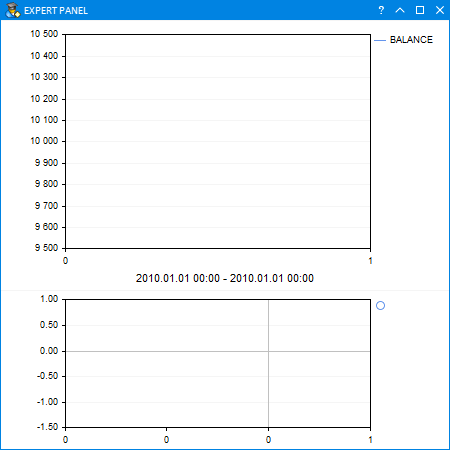### 来自信号服务的直观报告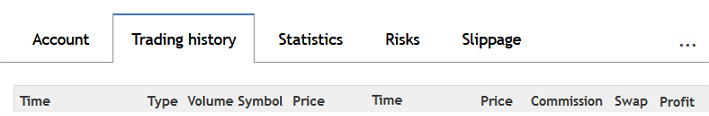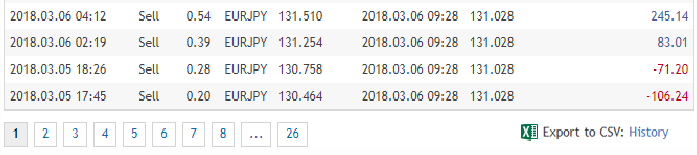```//+------------------------------------------------------------------+
//|                                                      Program.mqh |
//|                                版权所有 2018, MetaQuotes 软件公司 |
//|                                              http://www.mql5.com |
//+------------------------------------------------------------------+
//--- 外部参数
input string PathToFile=""; // 文件路径
...
```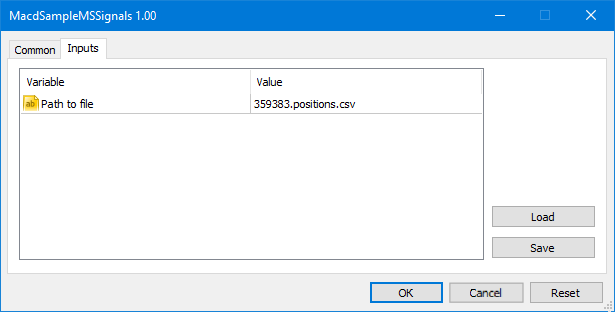```//--- 从文件中提取数据
struct CReportTable
{
string            m_rows[];
};
//+------------------------------------------------------------------+
//| 用于创建应用程序的类                                                 |
//+------------------------------------------------------------------+
class CProgram : public CWndEvents
{
private:
//--- 报表
CReportTable      m_columns[];
//--- 字符串和列的数量
uint              m_rows_total;
uint              m_columns_total;
};
//+------------------------------------------------------------------+
//| 构造器                                                            |
//+------------------------------------------------------------------+
CProgram::CProgram(void) : m_rows_total(0),
m_columns_total(0)
{
...
}
```

```class CProgram : public CWndEvents
{
private:
//--- 快速排序方法
void              QuickSort(uint beg,uint end,uint column);
//--- 检查排序条件
bool              CheckSortCondition(uint column_index,uint row_index,const string check_value,const bool direction);
//--- 在指定单元格中交换数值
void              Swap(uint r1,uint r2);
};
```

```class CProgram : public CWndEvents
{
private:
//--- 获取数据到数组
int               GetData(void);
};
//+------------------------------------------------------------------+
//| 从报告中获取品种数据                                                 |
//+------------------------------------------------------------------+
int CProgram::GetData(void)
{
...
}
```

```...
//--- 获取标题字符串元素
string str_elements[];
ushort u_sep=::StringGetCharacter(";",0);
::StringSplit(m_source_data,u_sep,str_elements);
//--- 字符串和字符串元素的数量
int strings_total  =::ArraySize(m_source_data);
int elements_total =::ArraySize(str_elements);
//--- 获取品种
if((m_symbols_total=GetHistorySymbols())==WRONG_VALUE)
return;
//--- 释放数组
::ArrayFree(m_dd_y);
::ArrayFree(m_dd_x);
//--- 数据序列大小
::ArrayResize(m_columns,elements_total);
for(int i=0; i<elements_total; i++)
::ArrayResize(m_columns[i].m_rows,strings_total-1);
//--- 用文件中的数据填充数组
for(int r=0; r<strings_total-1; r++)
{
::StringSplit(m_source_data[r+1],u_sep,str_elements);
for(int c=0; c<elements_total; c++)
m_columns[c].m_rows[r]=str_elements[c];
}
...
```

```...
//--- 序列和列的数量
m_rows_total    =strings_total-1;
m_columns_total =elements_total;
//--- 按第一列中的时间排序
QuickSort(0,m_rows_total-1,0);
//--- 序列大小
::ArrayResize(m_symbol_balance,m_symbols_total);
for(int i=0; i<m_symbols_total; i++)
::ArrayResize(m_symbol_balance[i].m_data,m_rows_total);
...
```

```...
//--- 余额和最大回撤
double balance      =0.0;
double max_drawdown =0.0;
//--- 获取总余额数据
for(uint i=0; i<m_rows_total; i++)
{
//--- 初始余额
if(i==0)
{
balance+=(double)m_columns[elements_total-1].m_rows[i];
m_symbol_balance.m_data[i]=balance;
}
else
{
//--- 跳过追加资金
if(m_columns.m_rows[i]=="Balance")
m_symbol_balance.m_data[i]=m_symbol_balance.m_data[i-1];
else
{
balance+=(double)m_columns[elements_total-1].m_rows[i]+(double)m_columns[elements_total-2].m_rows[i]+(double)m_columns[elements_total-3].m_rows[i];
m_symbol_balance.m_data[i]=balance;
}
}
//--- 计算回撤
if(MaxDrawdownToString(i,balance,max_drawdown)!="")
AddDrawDown(i,max_drawdown);
}
...
```

```...
//--- 获取品种余额数据
for(int s=1; s<m_symbols_total; s++)
{
//--- 初始余额
balance=m_symbol_balance.m_data;
m_symbol_balance[s].m_data=balance;
//---
for(uint r=0; r<m_rows_total; r++)
{
//--- 如果品种不匹配，则返回先前的值
if(m_symbols_name[s]!=m_columns[m_symbol_index].m_rows[r])
{
if(r>0)
m_symbol_balance[s].m_data[r]=m_symbol_balance[s].m_data[r-1];
//---
continue;
}
//--- 如果成交结果不为零
if((double)m_columns[elements_total-1].m_rows[r]!=0)
{
balance+=(double)m_columns[elements_total-1].m_rows[r]+(double)m_columns[elements_total-2].m_rows[r]+(double)m_columns[elements_total-3].m_rows[r];
m_symbol_balance[s].m_data[r]=balance;
}
//--- 否则，写入之前的值
else
m_symbol_balance[s].m_data[r]=m_symbol_balance[s].m_data[r-1];
}
}
...
```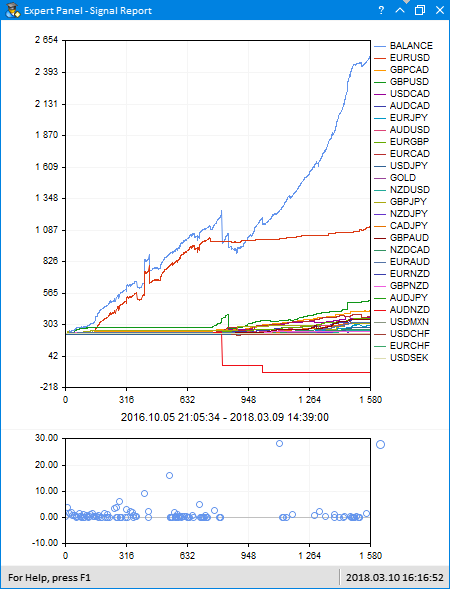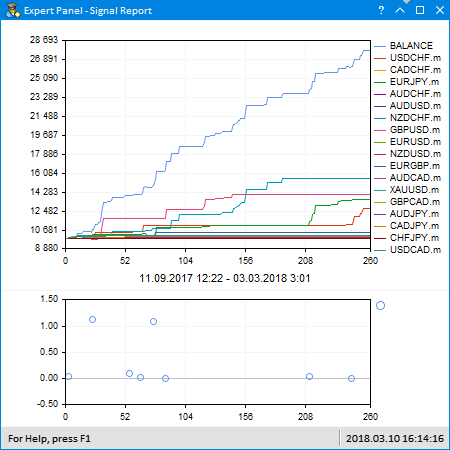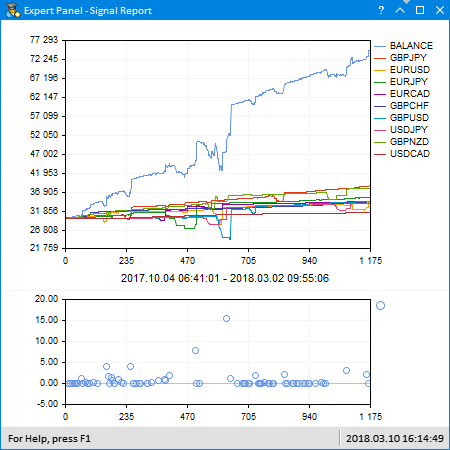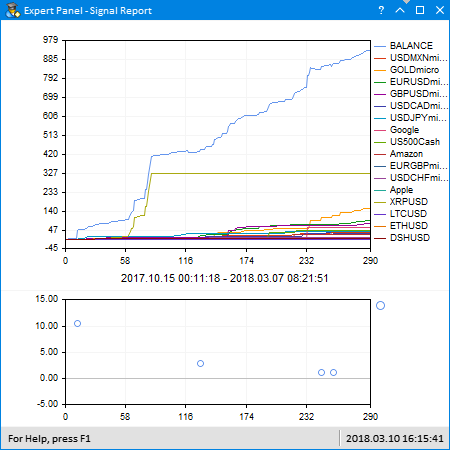### 结束语

MacdSampleMultiSymbols.mq5 自标准发行 MACD Sample 的改编版 EA
Program.mqh 程序类的文件
CreateGUI.mqh 文件实现来自 Program.mqh 文件的程序类方法
Strategy.mqh MACD Sample 策略类的改编文件 (多元品种版本)
FormatString.mqh 字符串格式化辅助函数的文件

MQL5.zip (39.77 KB)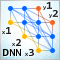深度神经网络 (第五部分)。 DNN 超参数的贝叶斯优化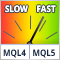自置缓存的指标速度比较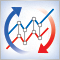同步多个相同交易品种而时段不同的图表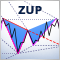ZUP - 通用之字折线 (ZigZag) 与 Pesavento 形态。 搜索形态

ZUP 指标允许搜索多个已知形态，其参数已经设置。 这些参数可以根据您的要求进行编辑。 您还可以使用 ZUP 图形界面创建新形态并将其参数保存到文件中。 之后，您可以快速检查这些新形态是否可以在图表中找到。